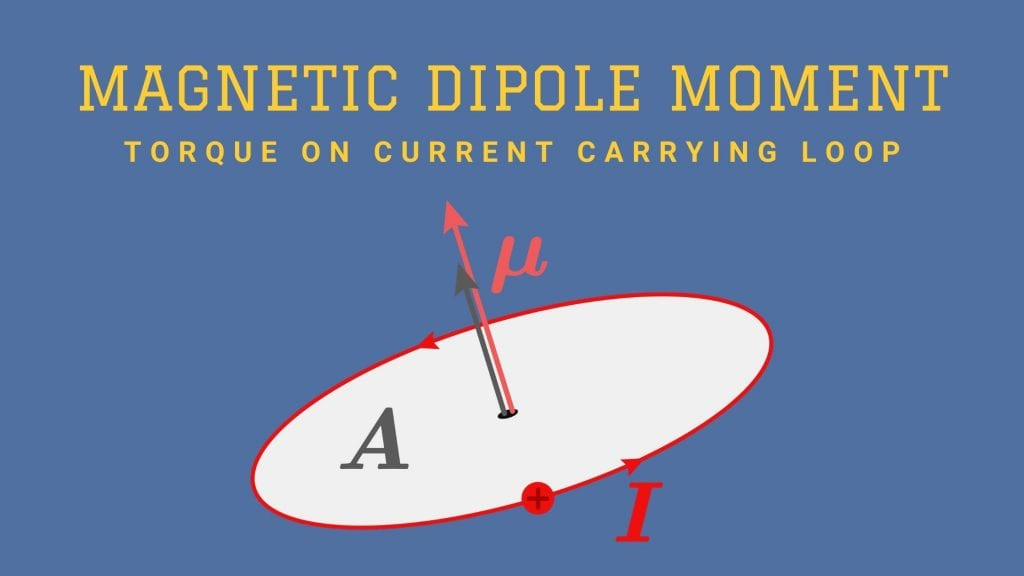Physics

# Magnetic Dipole Moment- Torque on Current Carrying Loop

A dipole is like a small magnet and magnetic moment of that dipole is called magnetic dipole moment. Read on magnetic dipole moment and related topics below.

4 minutes long
Posted by Mahak Jain, 7/6/2021Hesap Oluştur

Got stuck on homework? Get your step-by-step solutions from real tutors in minutes! 24/7. Unlimited.

## What is magnetic dipole moment in physics?

The magnetic dipole moment (µ) is a vector defined as multiplication of current and area vector whose direction is direction of area vector. Direction of area vector is determined by the right hand rule. Magnetic moment is also a name of magnetic dipole moment.

### Right hand thumb rule

Firstly, place your hand such that your thumb is perpendicular to plane of loop. Now, turn your fingers in the direction of current. Direction of thumb gives direction of resultant vector.

### Magnetic dipole moment formula

Formula of magnetic moment is, µ = iA, where i is current in the loop and A is area vector of that loop.

### Unit of dipole moment

SI unit of magnetic dipole moment = unit of i × unit of A = A m2.

### Direction of dipole moment

Direction of dipole moment is same as direction of area vector.

## Magnetic Dipole Moment of a Revolving Electron

The electron of charge (–e) (e = + 1.6 × 10-19 C) performs uniform circular motion around a stationary heavy nucleus of charge +Ze. We can write equivalent current I for it

I=e/T, where T is time period.

Let r be orbital radius and v be velocity.

⇒ T = 2πr/v ⇒ I = ev/2πr

Now, we know that magnetic dipole moment = µ = iA

⇒ µ = A × ev/2πr

µ = πr2 × ev/2πr = evr/2

Multiplying and dividing by me, mass of electron

⇒ µ = evrme / 2me

but mevr is angular momentum, l

µ = el / 2me

but µ = -el / 2me

The negative sign indicates that the angular momentum of the electron is opposite in direction to the magnetic moment.

### Gyromagnetic Ratio

Gyromagnetic ratio the ratio of magnetic dipole moment due to orbiting electron to its orbital angular momentum.

Thus, Gyromagnetic ratio = µ/l = e/2me

## Torque on Current Loop in Uniform Magnetic Field

Let’s consider a rectangular loop placed such that the uniform magnetic field B is in the plane of the loop as shown. Current in loop is I.

The magnetic field exerts no force on the two arms AD and BC of the loop because current in it makes 0° or 180° with magnetic field.

Since, magnetic field is perpendicular to the arm AB of the loop, it exerts a force F1 on it. F1 is directed into the plane of the loop.

Thus, F1 = BIb

Similarly, let force on arm CD be F2.

So, F2 = BIb

Thus, torque = τ = F1a/2 + F2a/2

⇒ τ = BIba/2 + BIba/2 = BI(ab)

τ = BIA, where A = ab is the area of rectangular loop.

When loop turns angle θ, τ = BIA sinθ.

Vector form of torque = τ = I(A × B), where A and B are vectors.

But we know that, IA=μ

τ = μ × B

Thus, magnetic dipole moment can also be defined as, a vector quantity which when cross multiplied by magnetic field vector gives torque.

## Circular Current Loop As a Magnetic Dipole

Here, we will see that the magnetic field (at large distances) due to current in a circular current loop is very similar in behavior to the electric field of an electric dipole.

Let us consider a circular loop of radius R carrying current I.

Thus, magnetic field on the axis of loop at distance x is

Note: if you don’t know how this formula came, then visit this blog.

When, x>>R, B = μ0IA/2πx3

Since, μ=IA

B = μ02m/4πx3